# Total internal reflection – slow to fast

When light travels from a medium such as glass to air, total internal reflection can occur.

Remember:Fast to slow, towards normal line

Slow to fast, away from normal line

Glass to air is slow to fast, therefore: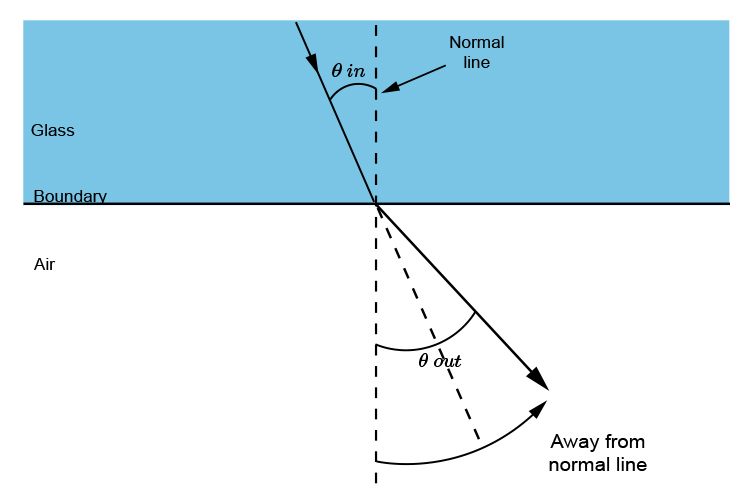It is at the point where theta\ \o\u\t=90^circ to the normal line that theta\ \i\n is at the critical angle.

The critical angle theta\ \i\n occurs when the light, theta\ \o\u\t, travels along the boundary between the two media.

The critical angle only occurs when travelling from a slow medium to a fast medium.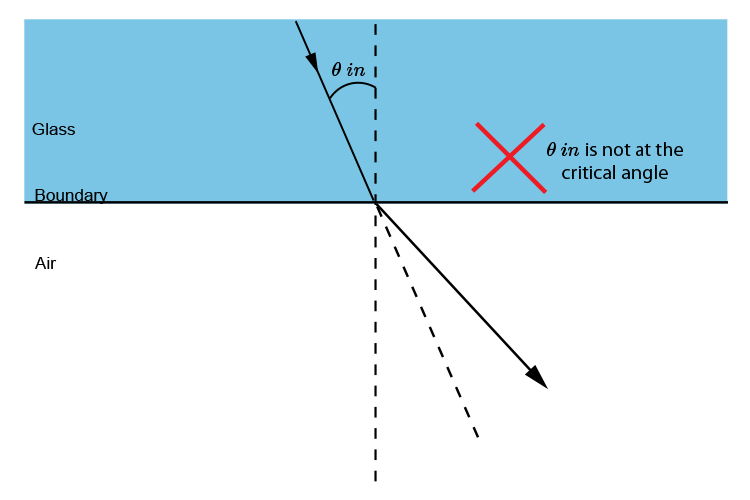As the angle of incidence is increased the angle of refraction increases.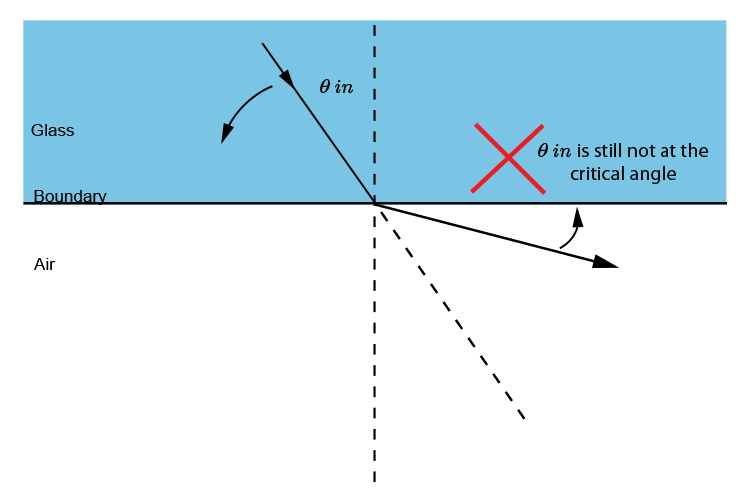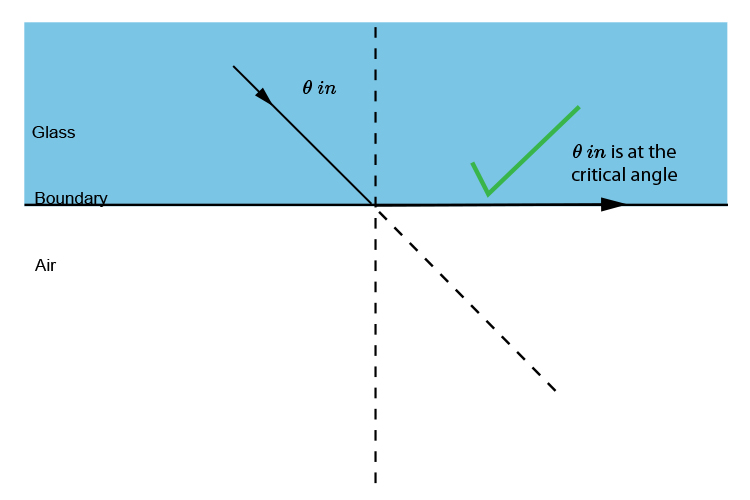At a certain angle (theta\ \i\n) the light will refract along the boundary. This is the critical angle for glass to air. It's worth knowing that (theta\ \i\n) is about 42 degrees for glass to air but it varies according to the material.

 Water to air ~~50^circ Glass to air ~~42^circ Diamond to air ~~24^circ

Past this point "total internal reflection" occurs.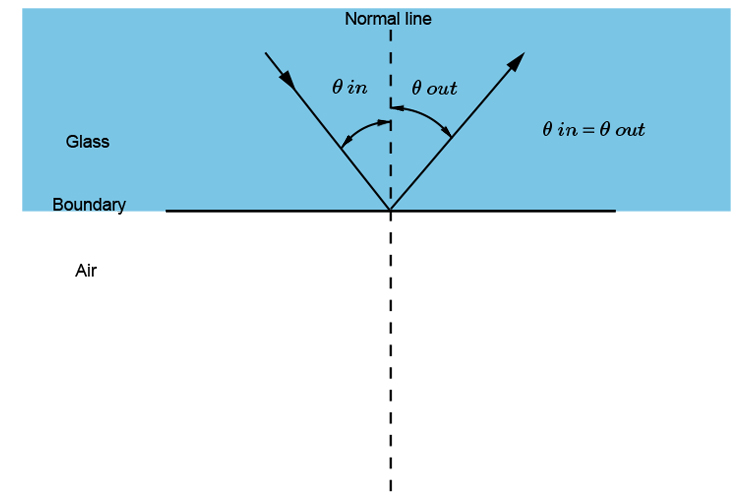It is not just a part of it that is reflected but all of the light ray gets reflected. This is why they call it total internal reflection.

Total internal reflection occurs when the angle of the light ray going in at angle (theta\ \i\n) is greater than the critical angle.

Total internal reflection theta\ \i\n\> critical angle